# Kinematics from Numerical Integration

Launch a particle from ground level with some speed and some angle and compute the trajectory using numerical integration. This is a very simple example for those learning to program.

  1 2 3 4 5 6 7 8 9 10 11 12 13 14 15 16 17 18 19 20 21 22 23 24 25 26 27 28 29 30 31 32 33 34 35 36 37 38 39 40 41 42 43 44 45 46 47 48 49 import math # Constants g = 10.0 # gravity v0 = 20.0 # initial speed theta0 = math.pi/4.0 # initial angle dt = 10.0**(-5.0) # time step ####################################### # Initialize simulation t = 0.0 # time count = 0 # count for printing x = 0.0 # initial position y = 0.0 xd = v0*math.cos(theta0) # initial velocity yd = v0*math.sin(theta0) xdd = 0.0 # initial acceleration ydd = -g ####################################### # Run simulation while y >= 0.0: # run while object in flight # numerical integration # explicit Euler x = x + xd*dt # update position based on velocity y = y + yd*dt xd = xd + xdd*dt # update velocity based on acceleration yd = yd + ydd*dt xdd = 0.0 # constant accleration ydd = -g t = t + dt # advance time and count count = count + 1 if count % 1000 == 0: # print once every thousand simulation intervals print t,x,y 

The results were plotted in Excel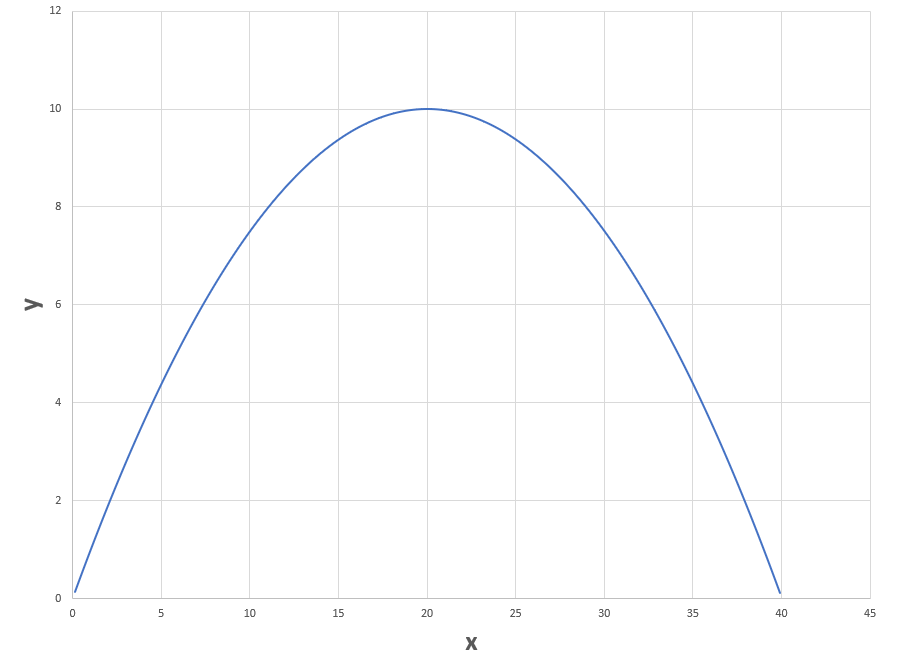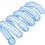Note by Steven Chase
4 months ago

This discussion board is a place to discuss our Daily Challenges and the math and science related to those challenges. Explanations are more than just a solution — they should explain the steps and thinking strategies that you used to obtain the solution. Comments should further the discussion of math and science.

When posting on Brilliant:

• Use the emojis to react to an explanation, whether you're congratulating a job well done , or just really confused .
• Ask specific questions about the challenge or the steps in somebody's explanation. Well-posed questions can add a lot to the discussion, but posting "I don't understand!" doesn't help anyone.
• Try to contribute something new to the discussion, whether it is an extension, generalization or other idea related to the challenge.

MarkdownAppears as
*italics* or _italics_ italics
**bold** or __bold__ bold
- bulleted- list
• bulleted
• list
1. numbered2. list
1. numbered
2. list
Note: you must add a full line of space before and after lists for them to show up correctly
paragraph 1paragraph 2

paragraph 1

paragraph 2

[example link](https://brilliant.org)example link
> This is a quote
This is a quote
    # I indented these lines
# 4 spaces, and now they show
# up as a code block.

print "hello world"
# I indented these lines
# 4 spaces, and now they show
# up as a code block.

print "hello world"
MathAppears as
Remember to wrap math in $$ ... $$ or $ ... $ to ensure proper formatting.
2 \times 3 $2 \times 3$
2^{34} $2^{34}$
a_{i-1} $a_{i-1}$
\frac{2}{3} $\frac{2}{3}$
\sqrt{2} $\sqrt{2}$
\sum_{i=1}^3 $\sum_{i=1}^3$
\sin \theta $\sin \theta$
\boxed{123} $\boxed{123}$

Sort by:

@Steven Chase thanks sir

@Steven Chase sir i have also typed the the whole code ,but my code is not expressed as your code is expressed in brilliant ??

You need three ticks and then "Python", and then code, followed by three more ticks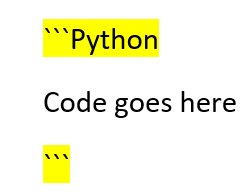- 4 months ago

  1 2 3 4 5 6 7 8 9 10 11 12 13 14 15 16 17 18 19 20 21 22 23 24 25 26 27 28 29 30 31 32 33 34 35 36 37 38 39 40 41 42 43 44 45 46 47 48 print "import math #constant g = 10.0 #gravity v0 = 20.0 #initial speed theta0 = math.pi/4.0 #initial speed dt = 10.0**(-5.0) #time step ########################################## #Initial simulation t = 0.0 #time count = 0 #count for printing x = 0.0 #initial position y = 0.0 xd = v0*math.cos(theta0) #initial velocity yd = v0*math.sin(theta0) xdd = 0.0 #initial acceleration ydd = -g ############################################## #Run simulation while y >= 0.0: #run while object in flight #numerical integration #elpicit euler x = x + xd*dt #update position based on velocity y = y + yd*dt xd = xd + xdd*dt #update velocity based on acceleration yd = yd + ydd*dt xdd = 0.0 #constanrt acceleration t = t + dt #advance time and count count = count + 1 if count % 1000 == 0: #print once every thousand simulation intervals print (t,x,y)" 

@Steven Chase sir can you please show a photo of your excel ,i am facing a bit of difficulty while plotting it in excel.

You have to import the data from a text file and choose space-delimited format

- 4 months ago

@Steven Chase i stucked here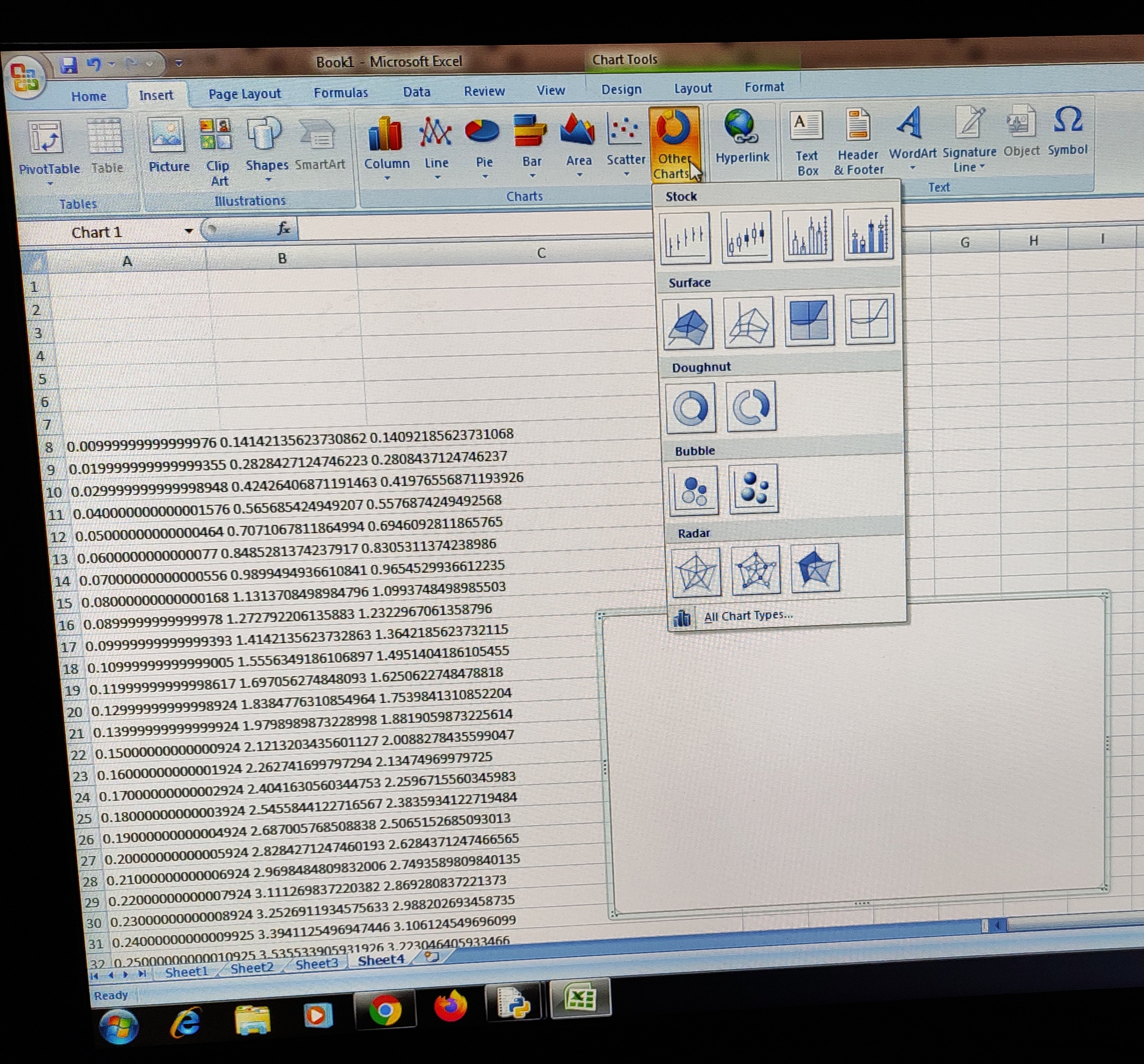Have have to import the data in space delimited format, which you haven't done. Then highlight columns B and C.

Insert - Scatter - Scatter with smooth lines

- 4 months ago

@Steven Chase which option should I choose here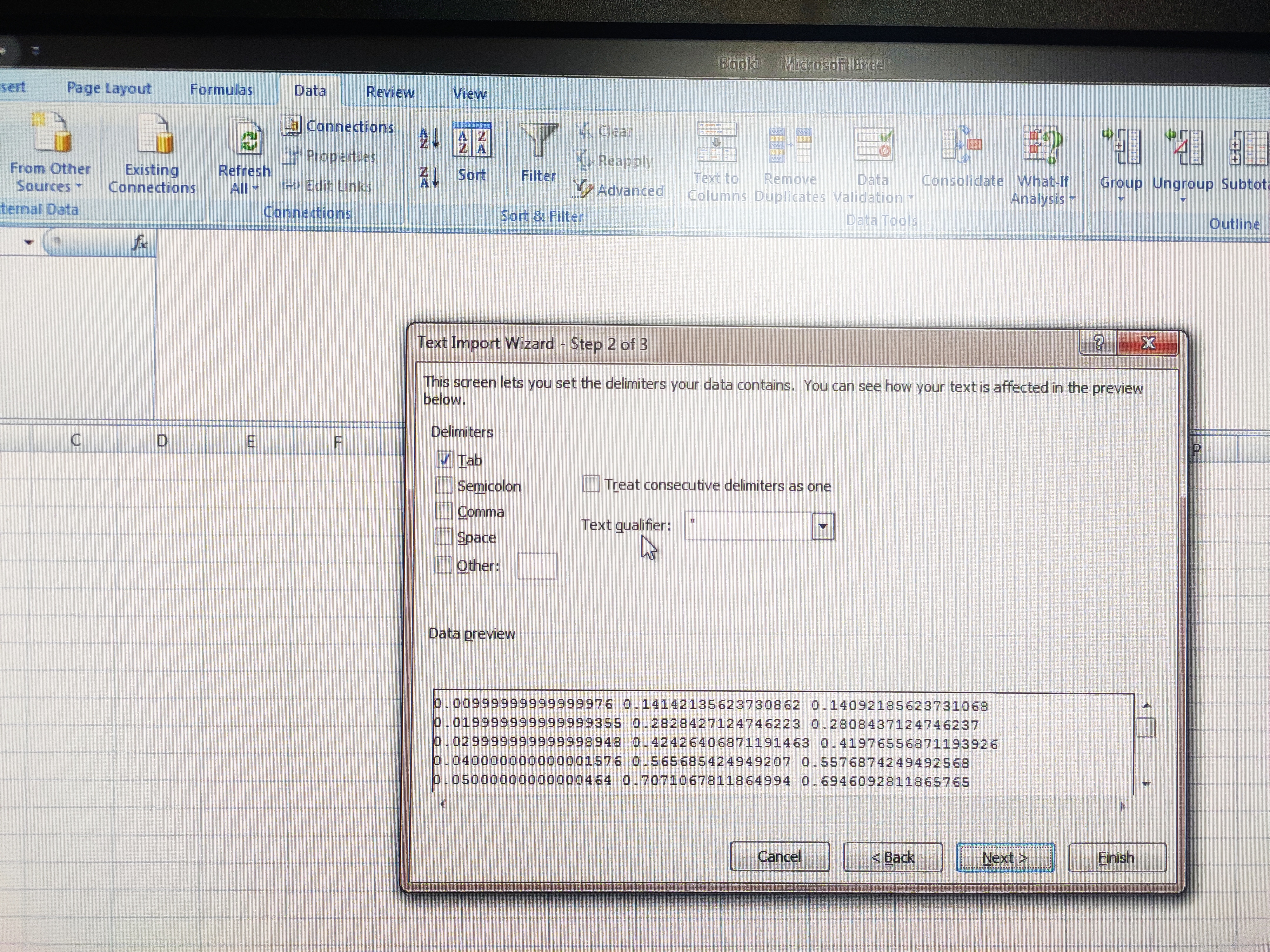space delimited

- 4 months ago

@Steven Chase which option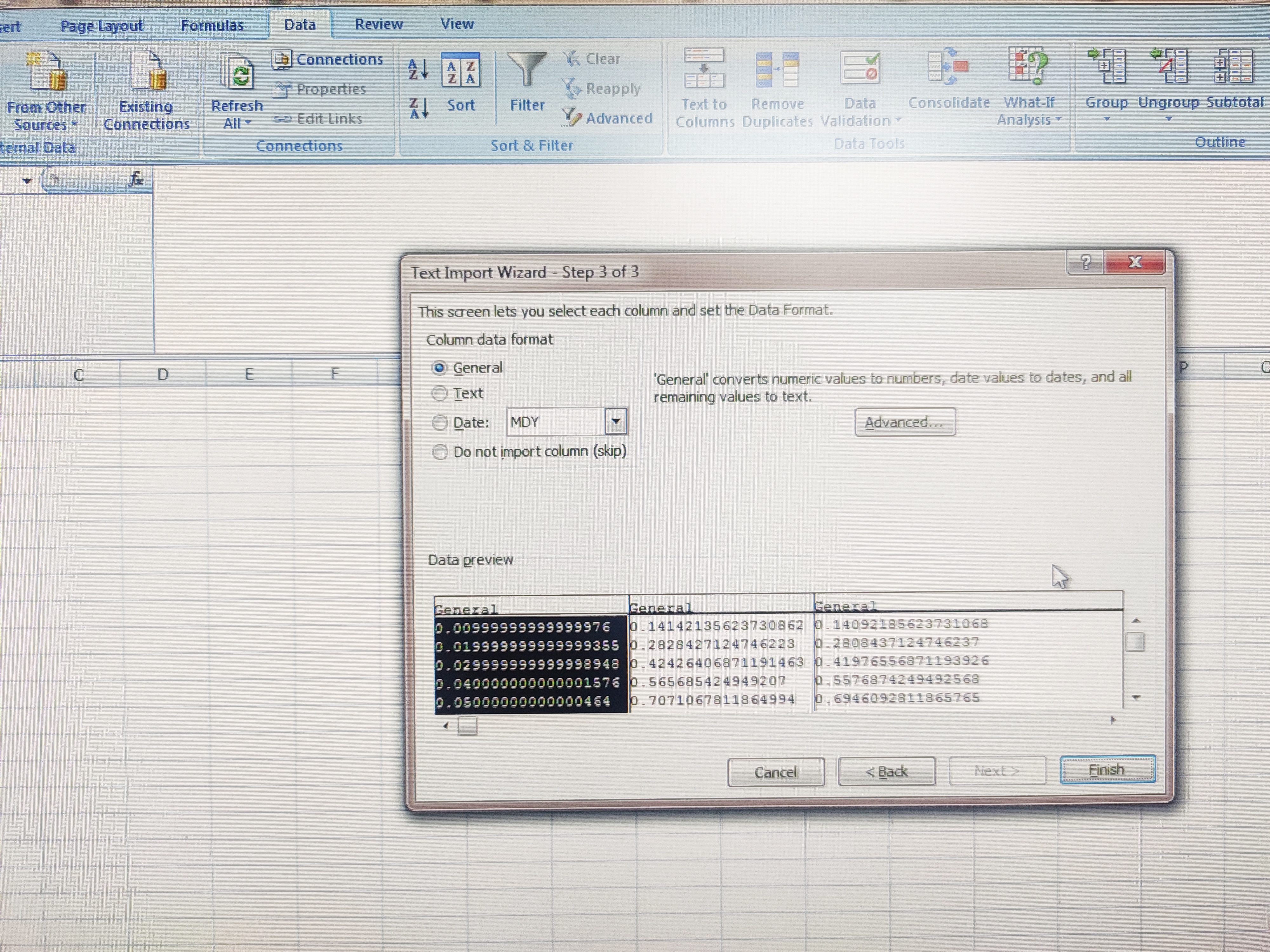Just hit finish

- 4 months ago

@Steven Chase now where I have to go?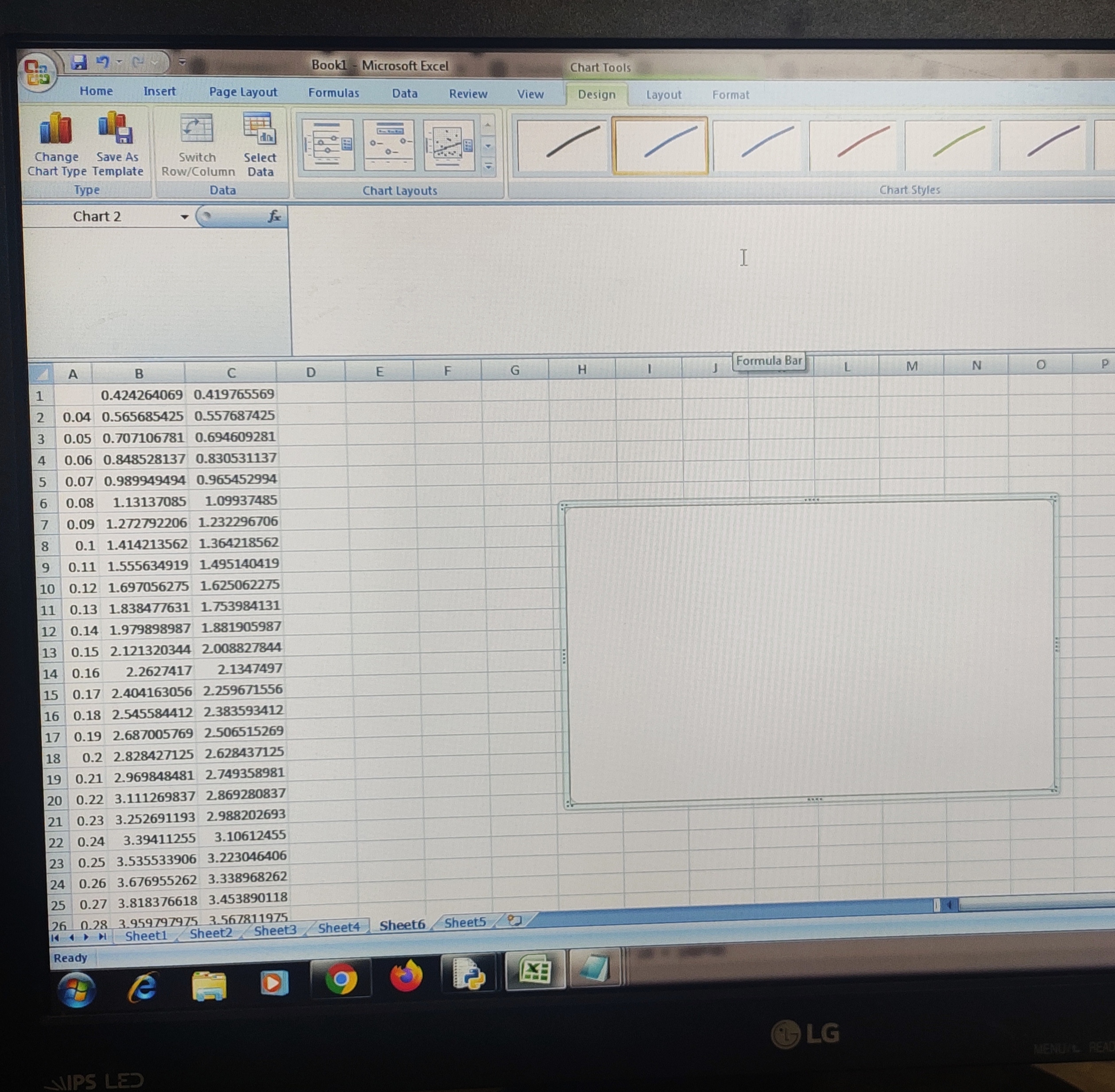Highlight columns B and C and then go to:

Insert - scatter chart - scatter with smooth lines

- 4 months ago

I was going to that scatter only by mistake my arrow is at (other charts)

@Steven Chase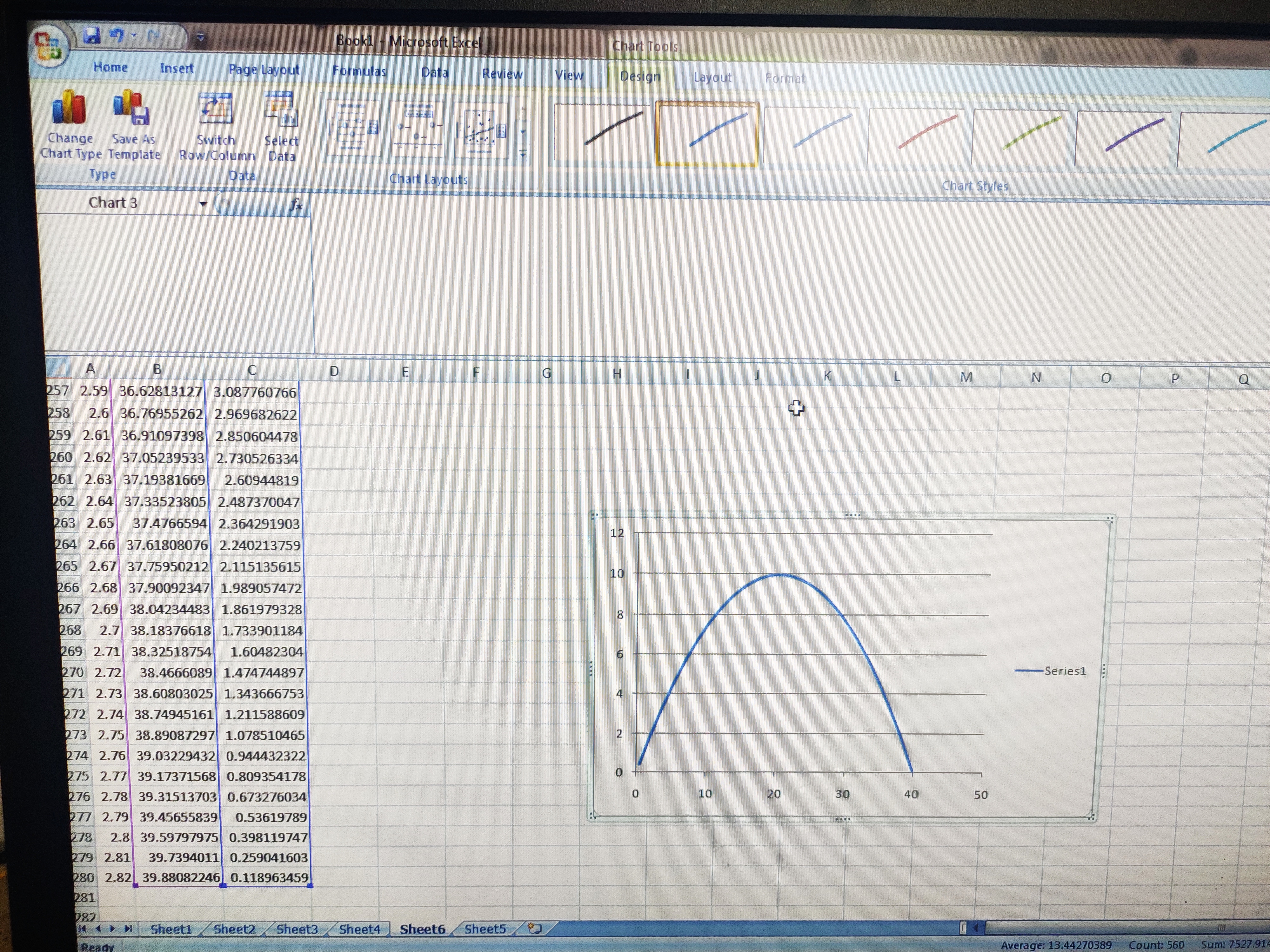There it is

- 4 months ago

@Steven Chase yes, Thank you sir
I don't think that anyone in the universe will help me like this you have helped me.

You're welcome. And the cool part is that nowhere in the code is any parabola explicitly specified or coded. It just comes out of the numerical integration

- 4 months ago

@Steven Chase yeah it is interesting

@Steven Chase sir in the last 2nd step what is the meaning of that double equal to = = ??

I single equals sign is used to declare the value of a quantity. A double equals sign is used to compare two quantities to see if they are equal

- 4 months ago

@Steven Chase okay! thanks , can you please give me some questions or post for practicing numerical integration .
if you post it should be of E and M.

Wow you're such a dedicated student. Really awesome to see passion and dedication :)

- 3 months, 4 weeks ago

@Steven Chase I want to learn monte Carlo, how can I learn??

Hey! You can check out this book.

- 4 months ago